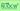# Econometrics IILecturer Hidehiko KAMIYA, Professor School of Economics / Graduate School of Economics, 2012 Spring Economics students (2・週1回全15回)

Econometrics is concerned with statistical methods for analyzing economic phenomena based on economic theory and real data. This course is designed for second-year undergraduate students who already have basic knowledge of regression analysis. The course covers further topics such as the violation of classical assumptions, simultaneous equations models, and time series analysis. It is intended that the students will acquire the fundamental ability to analyze economic phenomena using real data.

### Key Features

Numerical exercises are provided which require students to use a computer to analyze real data after each lecture. These exercises are reviewed in the next lecture, and the answers will also be available later at the course website. The intention is that the numerical exercises will supplement the lectures which are mainly focused on the theoretical aspect.

### Course Overview

Econometrics is concerned with statistical methods for analyzing economic phenomena based on economic theory and real data. This course is designed for second-year undergraduate students who already have basic knowledge of regression analysis. The course covers further topics such as the violation of classical assumptions, simultaneous equations models, and time series analysis. It is intended that the students will acquire the fundamental ability to analyze economic phenomena using real data.

### Course Requirements

The course prerequisites are "Statistical Analysis" and "Econometrics I". Familiarity with introductory statistics and fundamental regression analysis is assumed.

### Course Schedule

Session Contents
1 Orientation
2 Review of "Econometrics I"
3 Dummy variables (1)
4 Dummy variables (2)
5 The Gauss-Markov theorem
6 Regression models without the intercept
7 Heteroskedasticity (1)
8 Heteroskedasticity (2)
9 Serial correlation (1)
10 Serial correlation (2)
11 Serial correlation (3)
12 Simultaneous equations models
13 Other topics (1)
14 Other topics (2)
15 Summary / Final exam